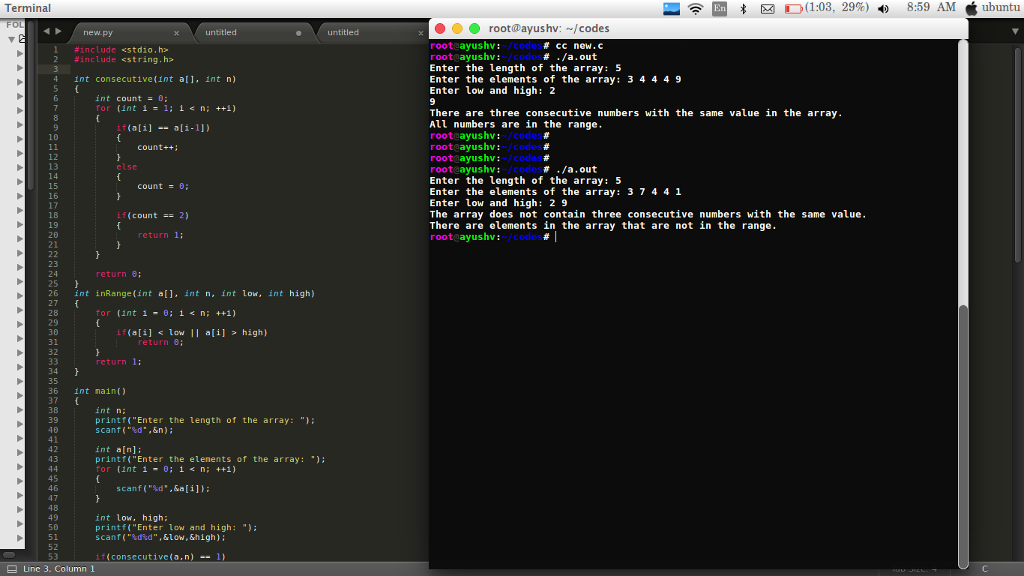# Answered! Program using C. Write a program range.c that include the following functions. int consecutive(int a[], int n);…

Program using C.

Write a program range.c that include the following functions.

Don't use plagiarized sources. Get Your Custom Essay on
Answered! Program using C. Write a program range.c that include the following functions. int consecutive(int a[], int n);…
GET AN ESSAY WRITTEN FOR YOU FROM AS LOW AS \$13/PAGE

int consecutive(int a[], int n);

int inRange(int a[], int n, int low, int high);

The consecutive function tests whether the integer array has three consecutive numbers with the same value. The function returns 1 if there are three consecutive numbers with the same value and return 0 if not. Assumes that the array has at least three elements;

The inRange function compares elements of the array with low and high, returns 1 if all the elements of the array are in the range (inclusive) and returns 0 if there is element in the array that are not in the range.

In the main function, ask the user to enter the length of the array, declare the array with the length, read in the values for the array and low and high, and call the two functions. The main function should display the result.

Sample run #1:

Enter the length of the array: 5

Enter the elements of the array: 3 4 4 4 9

Enter low and high: 2 9

Output:

There are three consecutive numbers with the same value in the array.

All numbers are in the range.

Sample run #2:

Enter the length of the array: 5

Enter the elements of the array: 3 7 4 4 1

Enter low and high: 2 9

Output:

The array does not contain three consecutive numbers with the same value.

There are elements in the array that are not in the range.

// C code

#include <stdio.h>
#include <string.h>

int consecutive(int a[], int n)
{
int count = 0;
for (int i = 1; i < n; ++i)
{
if(a[i] == a[i-1])
{
count++;
}
else
{
count = 0;
}

if(count == 2)
{
return 1;
}
}

return 0;
}
int inRange(int a[], int n, int low, int high)
{
for (int i = 0; i < n; ++i)
{
if(a[i] < low || a[i] > high)
return 0;
}
return 1;
}

int main()
{
int n;
printf(“Enter the length of the array: “);
scanf(“%d”,&n);

int a[n];
printf(“Enter the elements of the array: “);
for (int i = 0; i < n; ++i)
{
scanf(“%d”,&a[i]);
}

int low, high;
printf(“Enter low and high: “);
scanf(“%d%d”,&low,&high);

if(consecutive(a,n) == 1)
{
printf(“There are three consecutive numbers with the same value in the array.n”);
}
else
{
printf(“The array does not contain three consecutive numbers with the same value.n”);
}

if(inRange(a,n,low,high) == 1)
{
printf(“All numbers are in the range.n”);
}
else
{
printf(“There are elements in the array that are not in the range.n”);
}

return 0;
}